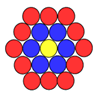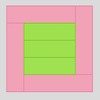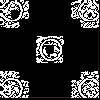#### You may also like### Counting Counters

Take a counter and surround it by a ring of other counters that MUST touch two others. How many are needed?### Cuisenaire Squares

These squares have been made from Cuisenaire rods. Can you describe the pattern? What would the next square look like?### Doplication

We can arrange dots in a similar way to the 5 on a dice and they usually sit quite well into a rectangular shape. How many altogether in this 3 by 5? What happens for other sizes?

# Dotty Circle

##### Age 7 to 11Challenge Level

Pupils from Stradbroke Primary School sent in some well-thought out solutions to this problem. Roni explains how they worked out how much further (from $20^\circ$) the point would have to turn to finish the same height above or below the horizontal axis:

1. A whole circle is $360^\circ$, half a circle is $180^\circ$.$180^\circ$ take away $20^\circ$ and then take away another $20^\circ$ gives the answer of $140^\circ$.
2. The first $20^\circ$ is above the x line and the third $20^\circ$ is under the x line so it's always $180^\circ$.
3. A whole circle is $360^\circ$, you take away $20^\circ$ and then another $20^\circ$. 20 add 20 equals 40. 360 take away 40 equals $320^\circ$.

So, Roni has concluded that there are three other points where the dot would be the same distance from the horizontal axis as it is for $20^\circ$. Another way of looking at it would be to say that the dot itself would have to be at $20^\circ$, $160^\circ$, $200^\circ$and $340^\circ$.

George tells us how we can work out how far a point at any position on the circle would have to turn to finish the same height above or below the horizontal axis. He agrees that there will be three other solutions:

1. Double the first degree and take it away from $180^\circ$.
2. Add $180^\circ$ to the your starting degree.
3. Double the starting degree and take it away from $360^\circ$.

Well done!